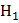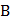# Resistancesandare joined in parallel and a current is passed so that the amount of heat liberated isandrespectively. The ratiohas the value a)b)c)d)## Question ID - 150227 :- Resistancesandare joined in parallel and a current is passed so that the amount of heat liberated isandrespectively. The ratiohas the value a)b)c)d)3537

 Two wiresandof same material and mass have their lengths in the ratio 1:2. On connecting them to the same source, the rate of heat dissipation inis found to beThe rate of heat dissipation inis a)b)c)d) None of these